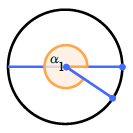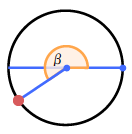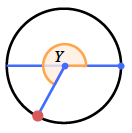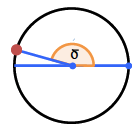### Home > PC3 > Chapter 2 > Lesson 2.2.4 > Problem2-105

2-105.

​​Sketch a unit circle. In your circle, sketch an angle between $0$ and $2\pi$ that has:

1. A positive cosine and a negative sine.2. A sine of $-0.5$.3. A negative cosine and a negative sine.4. A cosine of about $-0.9$ and a sine of about $0.3$.5. Can an angle have a sine equal to $0.9$ and cosine equal to $0.8$? Give an angle or explain why not.

Does $\sin^2(θ)+\cos^2(θ)=1$?